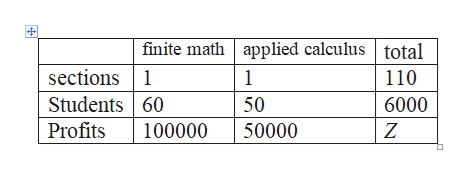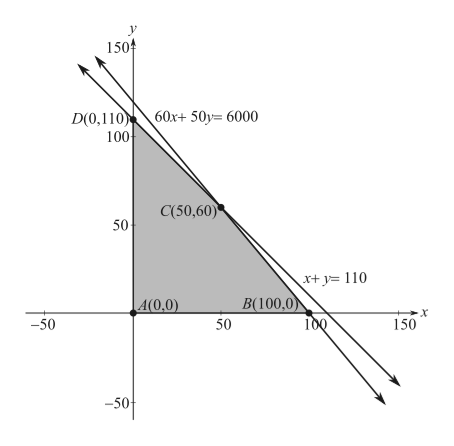# Podunk Insititute of Technology's Math Department offers two courses: Finite Math and Applied Calculus.Each section of Finite Math has 60 students, and each section of Applied Calculus has 50. The Department is allowed to offer a total of up to 110 sections. Furthermore, no more than 6,000 students want to take a math course. (No student will take more than one math course.) Sppose the university makes a profit of \$100,000 on each section of Finite Math and \$50,000 on each section of Applied Calculus. How many sections of each course should the department offer to make the largest profit?

Question
1 views

Podunk Insititute of Technology's Math Department offers two courses: Finite Math and Applied Calculus.Each section of Finite Math has 60 students, and each section of Applied Calculus has 50. The Department is allowed to offer a total of up to 110 sections. Furthermore, no more than 6,000 students want to take a math course. (No student will take more than one math course.) Sppose the university makes a profit of \$100,000 on each section of Finite Math and \$50,000 on each section of Applied Calculus. How many sections of each course should the department offer to make the largest profit?

check_circle

Step 1

Let x and y denotes the number of sections of finite math and applied calculus. Then given problem can be formulated as,help_outlineImage Transcriptionclosefinite math applied calculus total sections 1 110 Students 60 6000 50 Profits 100000 50000 Z fullscreen
Step 2

That is,

Step 3

Sketch the region as...help_outlineImage Transcriptionclose150 D(0,110)60x+ 50y= 6000 100 C(50,60) 50 ry110 B(100,0 100 4(0,0) -50 150 50 -50t fullscreen

### Want to see the full answer?

See Solution

#### Want to see this answer and more?

Solutions are written by subject experts who are available 24/7. Questions are typically answered within 1 hour.*

See Solution
*Response times may vary by subject and question.
Tagged in

### Math